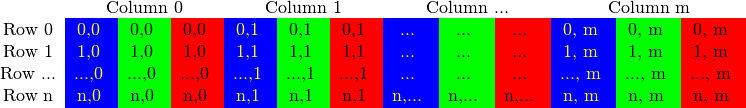# 在windows上安装opencv，并且创建visual studio 2017项目

## 安装

opencv的release页面下载opencv3.4.1的win pack。win pack是opencv适用于vs的免编译版本。

## 配置vs2017 创建项目

vs2017需要安装"使用c++的桌面开发"工作负载``````#include "stdafx.h"  //vs2017自定义
#include <opencv2/opencv.hpp>
#include<opencv2\core\core.hpp>
#include<opencv2\highgui\highgui.hpp>
using namespace cv;
using namespace std;
int main()
{
// 读入一张图片
// 创建一个名为 "photo"窗口
cvNamedWindow("photo");
Size dsize = Size(Image.cols*0.5, Image.rows*0.5);
Mat dst(dsize, Image.type());
resize(Image, dst, dst.size());

// 在窗口中显示游戏原画
imshow("photo", dst);
// 等待10000 ms后窗口自动关闭
waitKey(10000);
return 0;
}
``````

# core模块

## Mat

`Mat`这个类包含两部分信息：矩阵头信息和指向包含像素“值”的矩阵的指针。矩阵头信息包含：矩阵的大小、存储的方法、矩阵的地址等等。矩阵头信息中的矩阵大小是constant的，尽管矩阵本身大小会根据不同的图片改变。（mat代表一个图片，而mat由matrix实现，并且matrix会被复用。）

opencv是图像处理库，传递图像给函数是一种普遍的操作。下面讲讲将大大降低程序速度的行为——复制大图片（拷贝操作）。

``````Mat A, C;                          // creates just the header parts
A = imread(argv, IMREAD_COLOR); // here we'll know the method used (allocate matrix)
Mat B(A);                                 // Use the copy constructor
C = A;                                    // Assignment operator
``````

``````Mat D (A, Rect(10, 10, 100, 100) ); // using a rectangle
Mat E = A(Range::all(), Range(1,3)); // using row and column boundaries
``````

``````Mat F = A.clone();
Mat G;
A.copyTo(G);
``````

## matrix在内存中存储方式

RGB图像每列有三个值，分别记录B、G、R的值`Mat M(200, 200, CV_8UC3, Scalar(0, 0, 255));`这段代码地含义是创建一个`CV_8UC3`类型地宽200，高200的图片，BGR值分别为0，0，255，也就是红色。详细解释见下

Mat对象的type方法会返回图片的类型，最常见的图片类型是 16对应`CV_8UC3`。对应关系见下表。8UC3含义是用8位（也就是char）无符号地（U）表示像素值，所以像素值范围0-255。C3表示3 Channel（3个通道）。

C1 C2 C3 C4
CV_8U 0 8 16 24
CV_8S 1 9 17 25
CV_16U 2 10 18 26
CV_16S 3 11 19 27
CV_32S 4 12 20 28
CV_32F 5 13 21 29
CV_64F 6 14 22 30

## cv::LUT函数 遍历每个像素并改变其值

``````Parameters: 都是Mat类型

``````

``````Mat lookUpTable(1, 256, CV_8U);
uchar* p = lookUpTable.data;
for( int i = 0; i < 256; ++i)
p[i] = 255-i;

LUT(img,lut,img)
``````

## 操作图片

``````Mat img = imread(filename)//读取图片

imwrite(filename, img);//将图片写进文件，文件的格式取决于后缀。
``````

``````//注意y在前、x在后
//8UC1
Scalar intensity = img.at<uchar>(y, x);

//8UC3 最常用
Vec3b intensity = img.at<Vec3b>(y, x);
uchar blue = intensity.val;
uchar green = intensity.val;
uchar red = intensity.val;
``````

C1 C2 C3 C4 C6
uchar uchar cv::Vec2b cv::Vec3b cv::Vec4b
short short cv::Vec2s cv::Vec3s cv::Vec4s
int int cv::Vec2i cv::Vec3i cv::Vec4i
float float cv::Vec2f cv::Vec3f cv::Vec4f cv::Vec6f
double double cv::Vec2d cv::Vec3d cv::Vec4d cv::Vec6d

``````//8UC3
img.at<uchar>(y, x) = 128;
``````

``````Mat img = imread(".....");
cvNamedWindow("photo");//好像不是必须 另3.x是cvNamedWindow
imshow("photo", img);
waitKey();
``````

``````Size dsize = Size(Image.cols*0.5, Image.rows*0.5);
Mat dst(dsize, Image.type());
resize(Image, dst, dst.size());
``````

# vedeoio模块 调用摄像头、显示视频

``````#include "stdafx.h"
#include <opencv2/core.hpp>
#include <opencv2/videoio.hpp>
#include <opencv2/highgui.hpp>
#include <iostream>
#include <stdio.h>
using namespace cv;
using namespace std;
int main(int, char**)
{
Mat frame;
//--- INITIALIZE VIDEOCAPTURE
VideoCapture cap;
// open the default camera using default API
cap.open(0);
// OR advance usage: select any API backend
int deviceID = 0;             // 0 = open default camera
int apiID = cv::CAP_ANY;      // 0 = autodetect default API
// open selected camera using selected API
cap.open(deviceID + apiID);
// check if we succeeded
if (!cap.isOpened()) {
cerr << "ERROR! Unable to open camera\n";
return -1;
}
//--- GRAB AND WRITE LOOP
cout << "Start grabbing" << endl
<< "Press any key to terminate" << endl;
for (;;)
{
// wait for a new frame from camera and store it into 'frame'
// check if we succeeded
if (frame.empty()) {
cerr << "ERROR! blank frame grabbed\n";
break;
}
// show live and wait for a key with timeout long enough to show images
imshow("Live", frame);
if (waitKey(5) >= 0)
break;
}
// the camera will be deinitialized automatically in VideoCapture destructor
return 0;
}
``````

`cv::VideoCapture`这个类提供了进行视频操作的能力。这个本身依赖于FFmpeg开源库。video是由一连串的图片构成的，这些图片称之为帧（frame）。

``````//初始化
VideoWriter vw;     //新建一个多媒体文件
int fps = cap.get(CAP_PROP_FPS); //获取摄像头的帧率
if (fps <= 0)fps = 25;
//设置视频的格式
vw.open("E:\out.avi", VideoWriter::fourcc('M', 'J', 'P', 'G'), fps, Size(cap.get(CAP_PROP_FRAME_WIDTH), cap.get(CAP_PROP_FRAME_HEIGHT)));

//for循环中
vw.write(frame);   //将视频帧写入文件
``````

``````cv::VideoWriter::VideoWriter(
const String & 	filename,//文件名
int 	fourcc,//格式
double 	fps,//帧率
Size 	frameSize,//帧大小
bool 	isColor = true //是否彩色
)
``````